# Long short-term memory (LSTM) RNN in Tensorflow

Long short-term memory (LSTM) is an artificial recurrent neural network (RNN) architecture used in the field of deep learning. It was proposed in 1997 by Sepp Hochreiter and Jurgen schmidhuber . Unlike standard feed-forward neural networks, LSTM has feedback connections. It can process not only single data points (such as images) but also entire sequences of data (such as speech or video).

For example, LSTM is an application to tasks such as unsegmented, connected handwriting recognition, or speech recognition .

A general LSTM unit is composed of a cell, an input gate, an output gate, and a forget gate. The cell remembers values over arbitrary time intervals, and three gates regulate the flow of information into and out of the cell. LSTM is well-suited to classify, process, and predict the time series given of unknown duration.

Long Short- Term Memory (LSTM) networks are a modified version of recurrent neural networks, which makes it easier to remember past data in memory.

1. Input gate- It discover which value from input should be used to modify the memory. Sigmoid function decides which values to let through 0 or 1. And tanh function gives weightage to the values which are passed, deciding their level of importance ranging from -1 to 1 .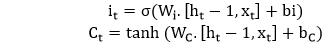2. Forget gate- It discover the details to be discarded from the block. A sigmoid function decides it. It looks at the previous state (ht-1) and the content input (Xt) and outputs a number between 0(omit this) and 1(keep this) for each number in the cell state Ct-1 .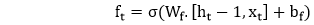3. Output gate- The input and the memory of the block are used to decide the output. Sigmoid function decides which values to let through 0 or 1. And tanh function decides which values to let through 0, 1. And tanh function gives weightage to the values which are passed, deciding their level of importance ranging from -1 to 1 and multiplied with an output of sigmoid .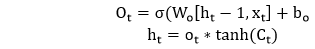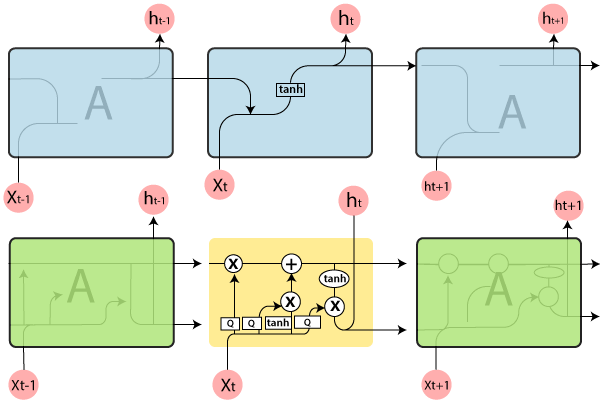It represents a full RNN cell that takes the current input of the sequence xi, and outputs the current hidden state, hi, passing this to the next RNN cell for our input sequence. The inside of an LSTM cell is a lot more complicated than a traditional RNN cell, while the conventional RNN cell has a single “internal layer” acting on the current state (ht-1) and input (xt).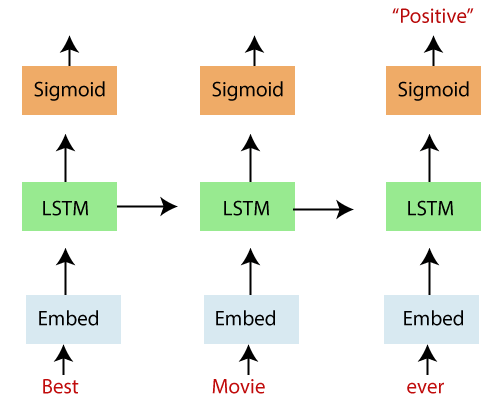In the above diagram, we see an “unrolled” LSTM network with an embedding layer, a subsequent LSTM layer, and a sigmoid activation function. We recognize that our inputs, in this case, words in a movie review, are input sequentially.

The words are inputted into an embedding lookup. In most cases, when working with a corpus of text data, the size of the vocabulary is unusually large.

This is a multidimensional, distributed representation of words in a vector space. These embeddings can be learned using other deep learning techniques like word2vec , we can train the model in an end-to-end fashion to determine the embedding as we teach.

These embeddings are then inputted into our LSTM layer , where the output is fed to a sigmoid output layer and the LSTM cell for the next word in our sequence.